# 浮点数表示

## —————————— 浮点数表示 ——————————

$$N = M\times R^E$$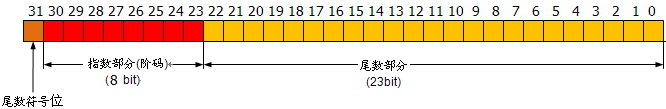## —————————— 浮点数的规格化表示 ——————————

$$0.3\times10^0$$；$$0.03\times10^1$$；$$0.003\times10^2$$；$$0.0003\times10^3$$；

(1) 尾数用原码,且隐藏尾数最高位。

(2) 阶码使用“移码”，基固定为2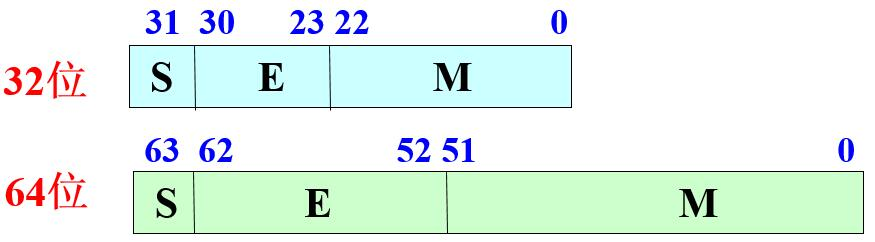$$x = (-1)^s\times(1.M)\times2^{E-127}$$

$$x = (-1)^s\times(1.M)\times2^{E-1023}$$

(1) 首先转化为2进制表示

$$-3.75 = -(2+1+1/2+1/4) = -1.111\times2^1$$

(2) 整理符号位并进行规格化表示

$$-1.111\times2^1 = (-1)^{(1)}\times(1+0.1110\ 0000\ 0000\ 0000\ 0000\ 000)\times2^1$$

(3) 进行阶码的移码处理
$$(-1)^{(1)}\times(1+0.1110\ 0000\ 0000\ 0000\ 0000\ 000)\times2^1$$
$$=(-1)^{(1)}\times(1+0.1110\ 0000\ 0000\ 0000\ 0000\ 000)\times2^{128-127}$$

$$1\ 1110\ 0000\ 0000\ 0000\ 0000\ 000\ 1000\ 0000$$

## —————————— 浮点数的表示范围 ——————————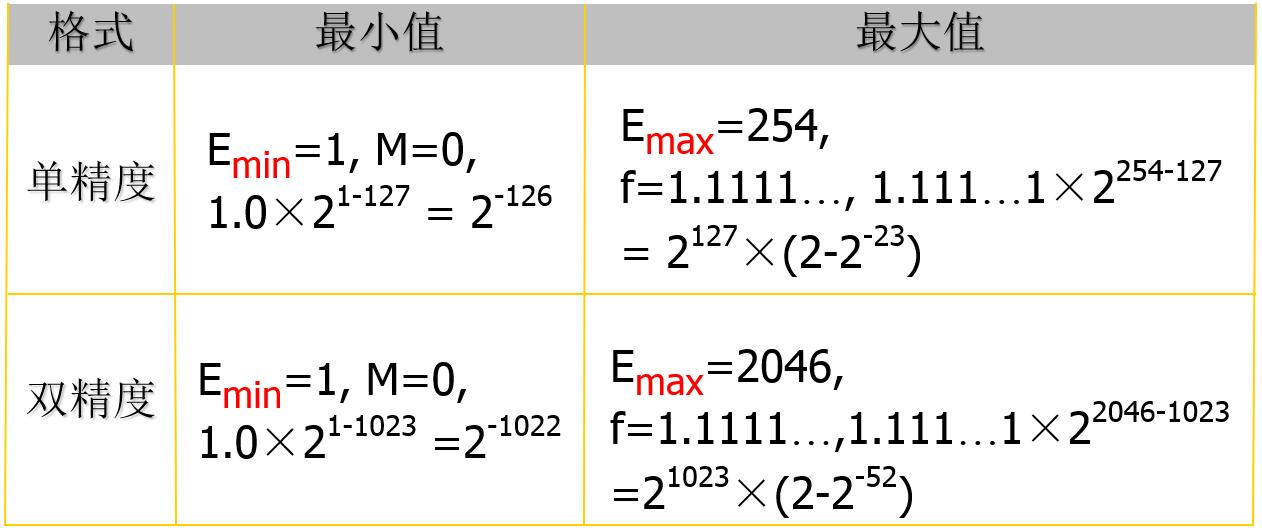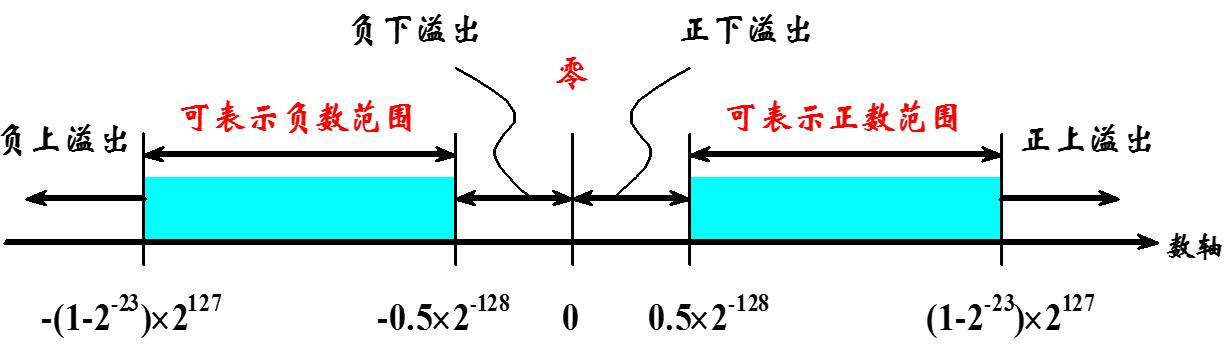(1)无穷值：

(+∞) + (+7) = (+∞)

(+∞) × (−2) = (−∞)

(+∞) × 0 = NaN

(2)零值：

(3)NAN：

## —————————— 浮点数的表示精度 ——————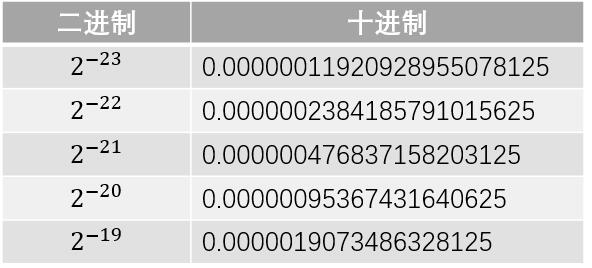$$2^{-23}$$ 和 $$2^{-22}$$ 之间是存在间隔的，即0.0000001和0.0000002之间的小数我们是没有办法描述的，因此23位尾数最多只能描述到小数点后第7位；此外，我们通过四舍五入可以很容易发现$$0.0000003 = 0.0000004 = 2^{-23}+2^{-22}$$, 这表明第7位有效数字只是部分准确。而第6位及之前的都是可以准确描述的，因此我们说float的有效位为6~7位。

## —————————— 参考资料 ——————————

(1) WIKI 词条 “Floating Point”: https://en.wikipedia.org/wiki/Floating_point

(2) WIKI 词条 “IEEE floating point”: https://en.wikipedia.org/wiki/IEEE_floating_point

(2) 浮点异常值：NAN，QNAN，SNAN:
http://www.cnblogs.com/konlil/archive/2011/07/06/2099646.html